# Locked Magnetic Island Chains

For the case of a locked magnetic island chain, we can set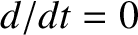in Equations (13.13) and (13.14). It follows that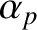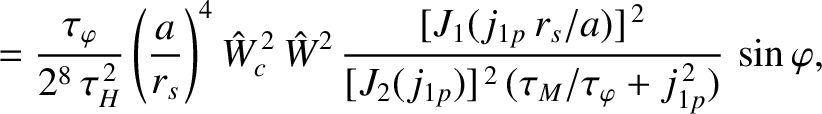(13.55)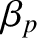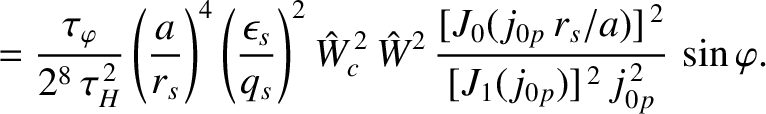(13.56)

Making use of Equations (7.34) and (7.35), we get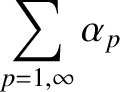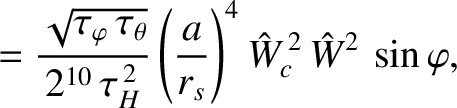(13.57)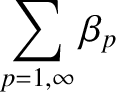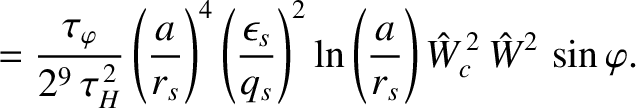(13.58)

Finally, settingin Equation (13.11), we obtain the torque balance criterion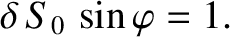(13.59)

Here, use has been made of Equations (13.29) and (13.46).

The left-hand side of the previous equation is the normalized electromagnetic locking torque that acts to maintain a fixed helical phase relation between the island chain and the static resonant magnetic perturbation, whereas the right-hand side is the normalized viscous torque that attempts to force the island chain to rotate with respect to the perturbation . In general, the previous equation possesses two solutions. However, only the solution in which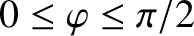is dynamically stable . Hence, we conclude that the island chain locks in a helical phase relative to the perturbation such that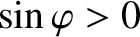and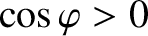, which is consistent with the numerical simulation shown in Figure 13.1.

Actually, the previous equation only possesses solutions when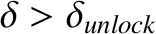, where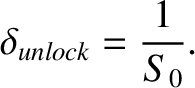(13.60)

If the locking parameter,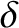, falls below the critical value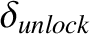then torque balance breaks down, and the island chain is forced to rotate with respect to the static resonant magnetic perturbation.

Now, making use of the data given in Table 13.2, we estimate that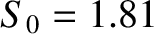for both a low-field and a high-field tokamak fusion reactor. It follows that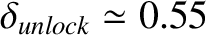in such reactors. According to Figure 13.2, this value is about half the critical value of the locking parameter above which a rotating magnetic island chain locks to a static resonant magnetic perturbation. In other words, once the locking parameter has exceeded the critical value required to cause a rotating island chain to lock to a static resonant magnetic perturbation, it must be reduced by a factor of about two before the island chain will unlock from the perturbation, and start to rotate again. This hysteresis between rotating and locked island states has been observed experimentally .

Finally, we deduce from Equations (13.29) and (13.60) and Table 13.2 that that the criterion for the unlocking of a rotating island chain from a static resonant magnetic perturbation is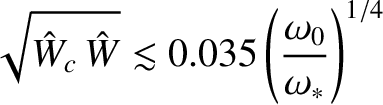(13.61)

in a low-field tokamak fusion reactor, and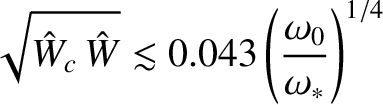(13.62)

in a high-field tokamak fusion reactor.